### Sample ProblemWhat is the voltage of C1 in the circuit above? V.

#### Solution

V =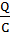C2 + 3 = 2.00F + 2.00F = 4.00 F=+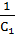=+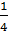=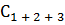= 2.00F

Qtotal = CV = 2.00F × 80.0V = 160.C

C1 is in series with the battery, so Q1 = Qtotal = 160.C

V ==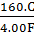= 40.0 V